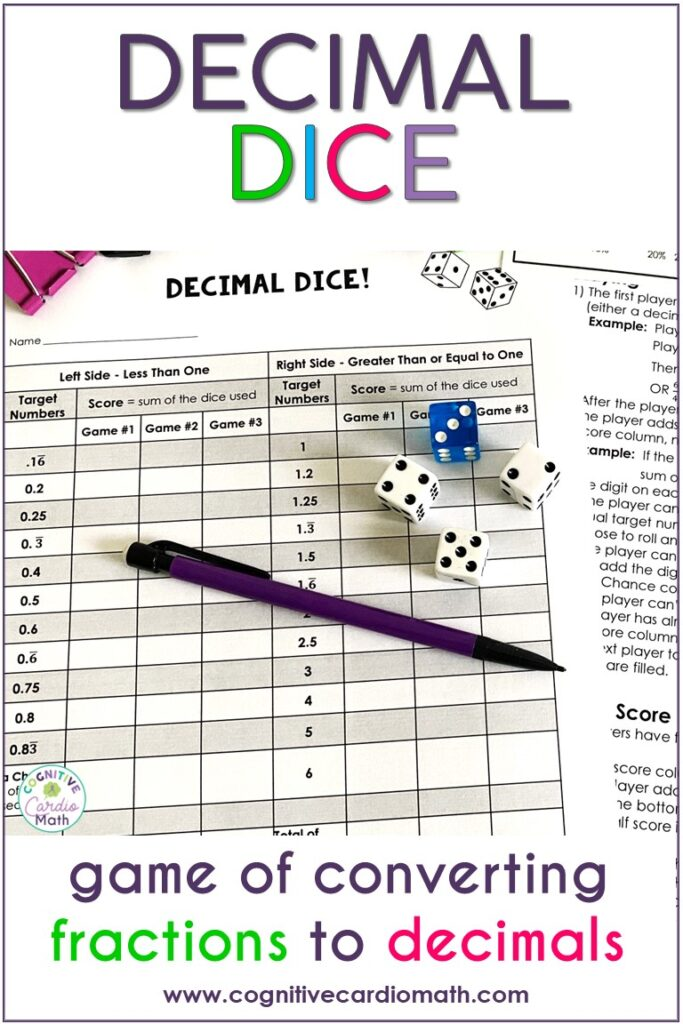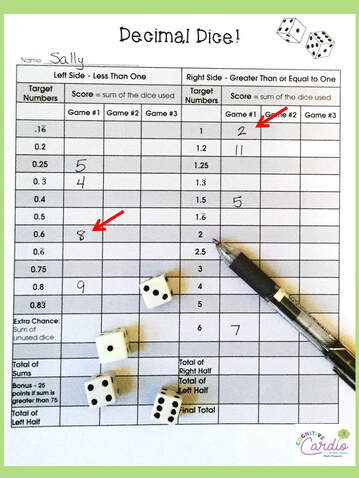# Converting Fractions to Decimals Activity ~ Playing "Decimal Dice"

## Challenging and Engaging Activity to Practice Fraction to Decimal Conversions

Could your math students use a little extra practice with converting fractions to decimals?
Do your middle school math students like to play math games? Mine sure do!

Over the past few years I’ve noticed that many of my math students aren’t familiar with some of the games I played when I was a kid, like Yahtzee, for example.So, as we started working on converting fractions and decimals, I decided to create a game to make fraction to decimal conversion practice more fun AND give them some more game experience! I based this fractions to decimals activity on the idea of Yahtzee:-)

## How the Converting Fractions to Decimals Game works (Example #1):

1) Students roll four dice, and pair the dice up to create fractions that equal “target numbers,” which are decimals or whole numbers.

For example, a student rolls 1, 2, 4, and 6. From these dice, the student may create any two of the following fractions, which convert to decimal (or whole) numbers:
½ = 0.5                    4/1 = 4
¼ = 0.25                  4/2 = 2
1/6 = 0.1666…           4/6 = 0.666…
2/1 = 2                        6/1 = 6
2/4 = 0.5                    6/2 = 3
2/6 = 0.333…             6/4 = 1.5

2) Once a player has chosen two target numbers, he or she finds their score by adding the dice that were used to get to each target number (decimal or whole number).
For example, if the player used  1 and 4 to get 0.25, he or she adds 1 + 4 for a sum of 5 to place in the score column. If the second target number used 2 and 6, to equal either 0.333…or 3, then sum of 8 would go in the appropriate column as the score.

## Converting Fractions to Decimals Game Example #2On the next roll, this student rolls 1, 1, 3, and 5. This student can:

• Pair 1 and 1, to get 1
• Pair 3 and 5 to get either 0.6 or 1.666…
• The score for 1 is 2 (1 + 1)
• The score for 0.6 (or 1.666) is 8 (3 + 5).

In many cases, students’ scores will be the same as each others’, but some of the decimals can be found with different combinations of numbers.
For example, 1 and 3 = 0.333…, and so do 2 and 6, so students could have a score of either 4 or 8 for this target  number.
Some students will notice this sum difference and go for the combination that will give them the higher score….bringing in the possibility of using some strategy, for those higher level thinkers.
The students have really enjoyed playing this fraction to decimal conversion game. They DO need a few examples at the start, to understand exactly how the game works, so if you decide to try the game, be prepared to go through a few turns together.

## Score Sheet

Detailed instructions are included in the Decimal Dice resource.
• A complete answer key of highest and lowest possible scores for each target number are included
• This is handy to quickly check student score cards as you check in on their games

If you give this converting fractions to decimals activity (Decimal Dice Game) a try, please let me know how it goes!

## EllieWelcome to Cognitive Cardio Math! I’m Ellie, a wife, mom, grandma, and dog ‘mom,’ and I’ve spent just about my whole life in school! With nearly 30 years in education, I’ve taught:

• All subject areas in 4th and 5th grades
• Math, ELA, and science in 6th grade (middle school)

I’ve been creating resources for teachers since 2012 and have worked in the elearning industry for about five years as well!